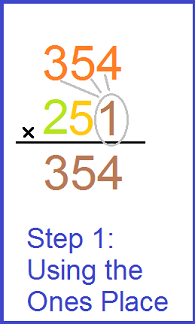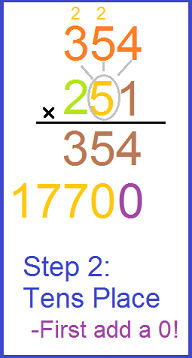Three Digit Multiplication

Instructor: Christine Quist

Christine has taught 4th-5th grade, has worked as a Paraprofessional for Adult Learners, and has a master's degree in Curriculum and Instruction for Elementary Education.

You've made it to the big leagues of multiplication! Once you've learned three-digit multiplication you can multiply any two numbers. This lesson will go over how to multiply with three-digit numbers.

Three Digit Numbers

Before we have fun multiplying three-digit numbers, let's make sure that we all know what a three-digit number is! The number 123 is a three-digit number. The digits in the number are 1, 2, and 3. The 1 is in the ones place, the 2 is in the tens place, and the 3 is in the hundreds place. A three-digit number is a number that has three digits. Easy, right?

How to Multiply

Let's learn how to multiply 354 by 251. The key idea when multiplying three-digit numbers is that every digit gets a turn to multiply with the other number's digits. Let's start by setting up our multiplication problem. Write the number 354 on the first row, and the number 251 on the second row. It is really important to make sure that the hundreds digits, tens digits, and ones digits are all lined up correctly!

Ones Digit

Let's start by multiplying the one's digit in the bottom number by each digit in the top number! Always start with the ones place. The one's digit in the bottom number is 1! So, we will multiply 1 with each of the digits in the top number.

Once each digit in the top number has a chance to multiply with the 1, you will be left with 354 on the first row.Tens Digit

Remember whenever we move to a new place value to start to multiply, you have to put the zeros down first. These zeros are your placeholders! You will write 1 zero for the tens and 2 zeros for the hundreds. This is because you are not just multiplying with a 5 in the tens place, you are actually multiplying with a 50! So, that 0 has to be present. You'll see this with the purple 0's in the pictures.

1. Start by putting a 0 in the ones place on the second row. This is the tens place row.
2. When you do 5 x 4 the answer is 20. This means you drop the 0 down below in the tens place and carry the 2.
3. This means 5 x 5 is 25 plus the 2 is 27. So, drop the 7 and carry the 2.
4. Do the same with 5 x 3 plus the 2 which equals 17. Since there are no more numbers to carry the 1 to, the 17 goes straight down to be in front of the second number on the tens place row.To unlock this lesson you must be a Study.com Member.

Register to view this lesson

Are you a student or a teacher?

See for yourself why 30 million people use Study.com

Become a Study.com member and start learning now.
Back
What teachers are saying about Study.com

Earning College Credit

Did you know… We have over 200 college courses that prepare you to earn credit by exam that is accepted by over 1,500 colleges and universities. You can test out of the first two years of college and save thousands off your degree. Anyone can earn credit-by-exam regardless of age or education level.